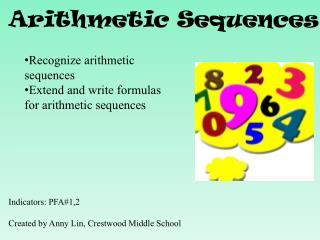DownloadDownload PresentationArithmetic Sequences

# Arithmetic Sequences

Download Presentation## Arithmetic Sequences

- - - - - - - - - - - - - - - - - - - - - - - - - - - E N D - - - - - - - - - - - - - - - - - - - - - - - - - - -
##### Presentation Transcript

1. Arithmetic Sequences • Recognize arithmetic sequences • Extend and write formulas for arithmetic sequences Indicators: PFA#1,2 Created by Anny Lin, Crestwood Middle School

2. A sequence is a set of numbers called terms, in a specific order.If the difference between successive terms is constant, then it is called arithmetic sequence.The difference between the terms is called the common difference.

3. You can use the common difference of an arithmetic sequence to find the next term in the sequence. Term: Arithmetic Sequence: Common Difference http://www.basic-mathematics.com/images/sequence1.gif

4. In other words, an arithmetic sequence is a numerical pattern that increases or decreases at a constant rate or value called the common difference. 3, 7, 11, 15,… 2, 6, 10, 14,… 13, 9, 5, 1,… 16, 12, 9, 8, 4, 2,… What about -17, -8, 3,14,25,…? This is Arithmetic. Arithmetic Not Arithmetic

5. Is the following sequence arithmetic? 0.7, 0.5, 0.3, 0.1,… • Yes • No The common difference is -0.2.

6. 3, 6, 12, 24,… -7, -3, 1, 5,... 1/5, 1/7, 1/9, 1/11,... -10, 5, -5/2, 5/4,... Which sequence is an arithmetic sequence?

7. -7, 0, 7, 14,… 0, 1/2, 1, 3/2,... 10, 6, 2, -2,... 2, 4, 8, 16,... Which sequence is NOT an arithmetic sequence?

8. Is the following sequence arithmetic? Yes No 1/2, 1/4, 1/8, 1/16,…

9. You can use the common difference of an arithmetic sequence to find the next term in the sequence.

10. Find the next three terms of the arithmetic sequence. • -15, -13, -11, -9,… What is the common difference? 2 What is the next term? -7 What are the next three terms? -7, -5, -3

11. Find the next three terms of the arithmetic sequence. 1/8, 1/4, 3/8 ,1/2 ,… What is the common difference? 1/8 What is the next term? 5/8 What are the next three terms? 5/8, ¾, 7/8

12. Real-World Example BOOKS The arithmetic sequence 36, 39, 42, 45, … represents the number of books in Jacob’s collection at the end of each month. Find the next three terms. Find the common difference by subtracting successive terms. The common difference is +3. Add 3 to the last term of the sequence to get the next term in the sequence. Continue adding 3 until the next three terms are found.

13. P 168 #1-4, 12-19 all • Worksheet for third period

14. Writing Arithmetic Sequence Words: Each term of an arithmetic sequence after the first term can be found by adding the common difference to the preceding term.

15. Symbols: An arithmetic sequence, a1 , a2 ,…, can be found as following: a1, a2 =a1 +d, a3 =a2 +d, a4 = a3 +d…, Where d is the common differences a1 is the first term, a2 is the second term, and so on.

16. Given the arithmetic sequence 3, 6, 9, 12, 15,… Find a1, a2, a3, a4. a1 =3 What is a5 ? a2 =6 a5 =15 What about a6 ? a3 =9 a4 =12 a6 =18 What is the common difference? 3

17. Given the arithmetic sequence 14, 28, 42, 56,… Find a1, a2, a3, a4. a1 =14 What is a5 ? a2 =28 a5 =70 What about a6 ? a3 =42 a4 =56 a6 =84 What is the common difference? 14

18. Given a1=6 and common differences: d=9. What is a2? a1=6 a2 =a1 +d =6+9 =15 a4 = a3 +d a3 =a2 +d =15+9 =24 =24+9 =33

19. For example: common differences: d=-8 a1=24 a2 =a1 +d =24-8 =16 a3 =a2 +d =16-8 =8 a4 = a3 +d =8-8 =0

20. 25, 23, 21, 19, …, What is the common difference d? What is a1 ? 25 d=-2 What is a2 ? 23 a2 =a1 +d =25+(-2) =23

21. 25, 23, 21, 19, …, d=-2 What is a3 ? What is a4 ? a4 =a3+d = a1 +d+d+d a4 = a1 +3d =25+3(-2) =25-6 =19 a3 =a2 +d = a1 +d+d a3= a1 +2d =25+2(-2) =25-4 =21

22. Watch Out!!! • Do you see the pattern??

23. What is the relationship between a3 and 2d? What is the relationship between a4 and 3d?

24. What is the relationship between an and (n-1)d?

25. d=2 25, 27, 29, 31, …, What is a4 ? What is an ? a4 =a3+ d = a2+ d+d = a1 + d+d+d a4 = a1 +3d =25+3(2) =25 + 6 =31 an =a1 +( )d = a1 +(n-1)d =25 +(n-1)(2)

26. Use the smart board

27. Formula The nth term an of an arithmetic sequence with first term a1 and common difference d is given by an =a1 +(n-1)d. Where n is a positive integer.